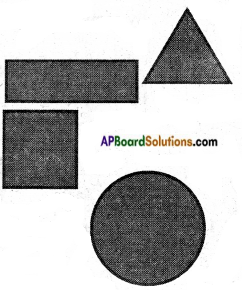Students can go through AP Board 6th Class Maths Notes Chapter 11 Perimeter and Area to understand and remember the concepts easily.

## AP State Board Syllabus 6th Class Maths Notes Chapter 11 Perimeter and Area

→ Perimeter: The perimeter of a polygon is sum of all its sides.
The perimeter of an equilateral triangle is P = 3 × side
The perimeter of a rectangle P = 2 (length + breadth)
And its area A = length × breadth A = l × b
The perimeter of a square is P = 4 × side And its area A = side × side (or)
A = s × s The circumference of a circle C = 2πr where r is the radius of the circle.→ Area: The region occupied by a plane figure is called its area.
To find the area of a complex figure, we divide the given shape into the combination of rectangles, squares and triangles where ever necessary.